# Tweets by @WolframResearch (Datasets for Multiparadigm Data Science Course)

These datasets are used in the example demonstrated in the "Build a Project Workflow" section of the "Multiparadigm Data Science" interactive course on Wolfram U

## Details

The following information is available for each tweet in the dataset: {ID, Text, Date, Hashtags, Location, Username, Name, ProfileImageThumbnail, RetweetCount, FavoriteCount, URL}

## Examples

### Basic Examples

Retrieve the resource:

 In:=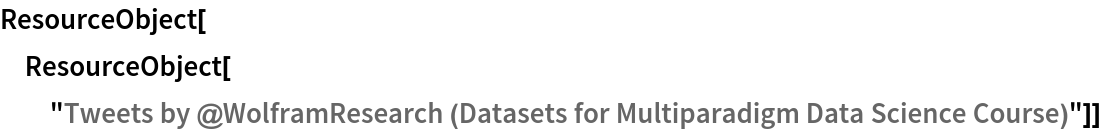Out=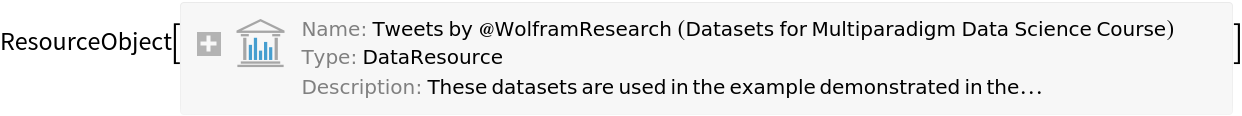Retrieve the default content::

 In:=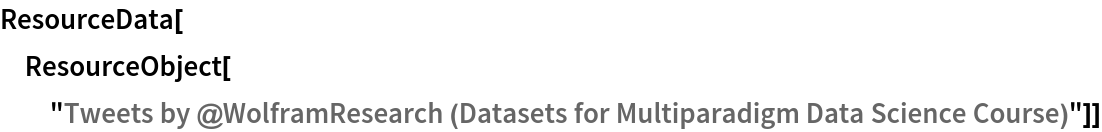Out=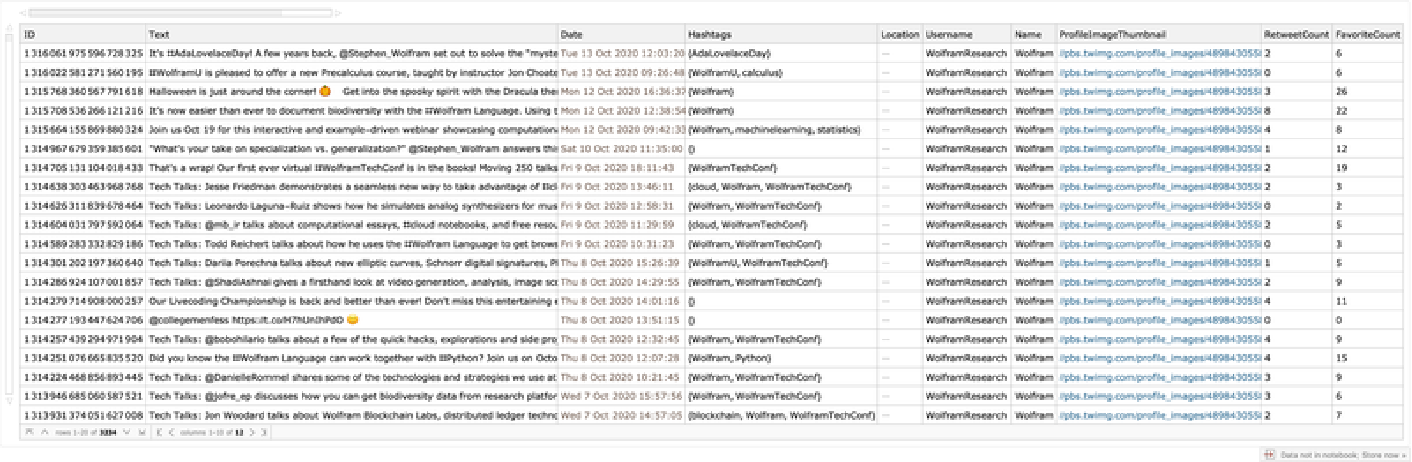Retrieve user data for the users mentioned in the tweets by WolframResearch:

 In:=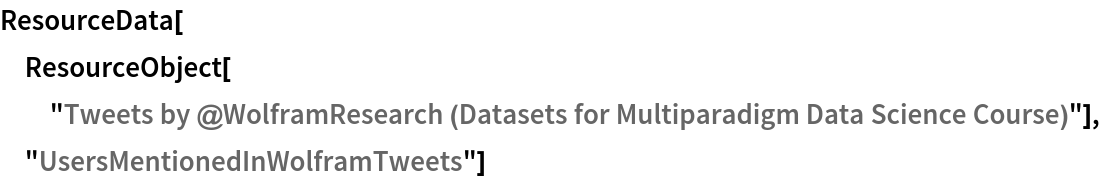Out=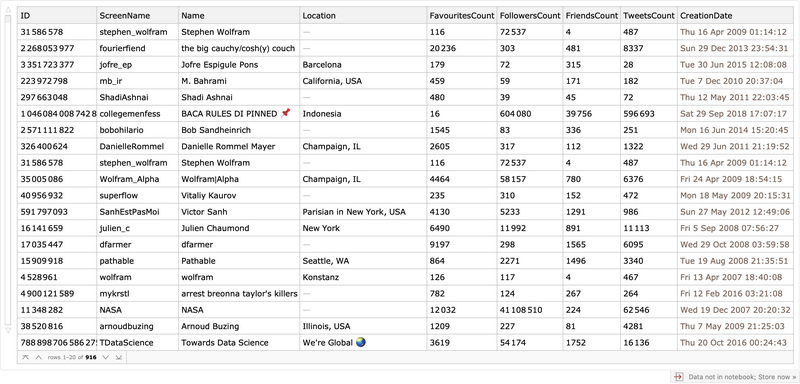Retrieve locations of the users mentioned in the tweets by WolframResearch (3 random locations):

 In:=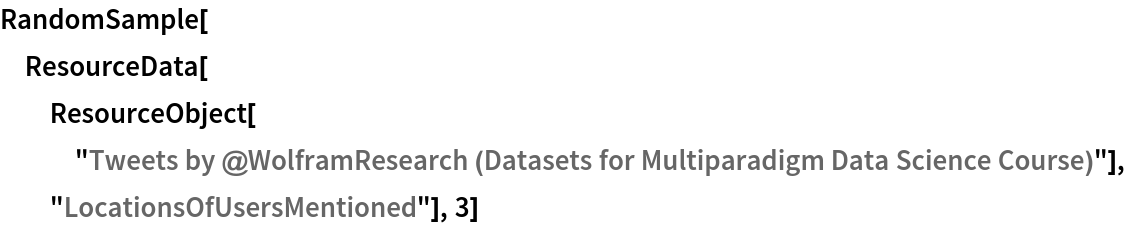Out=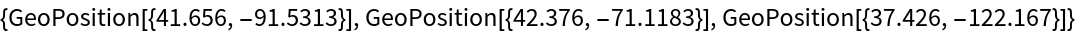Retrieve hashtags used by the users mentioned in the tweets by WolframResearch (3 random users):

 In:=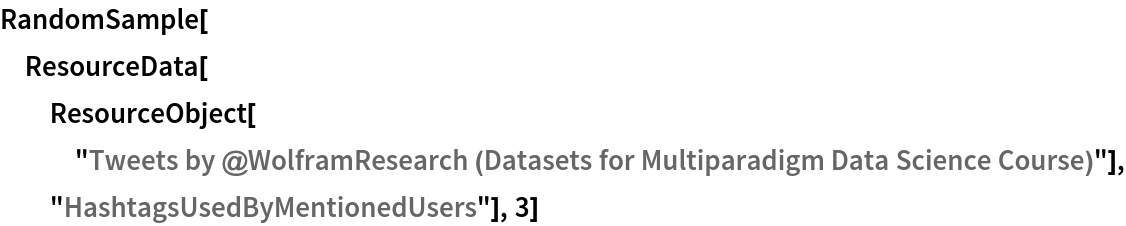Out=Retrieve a graph of users mentioning each other (3 random edges):

 In:=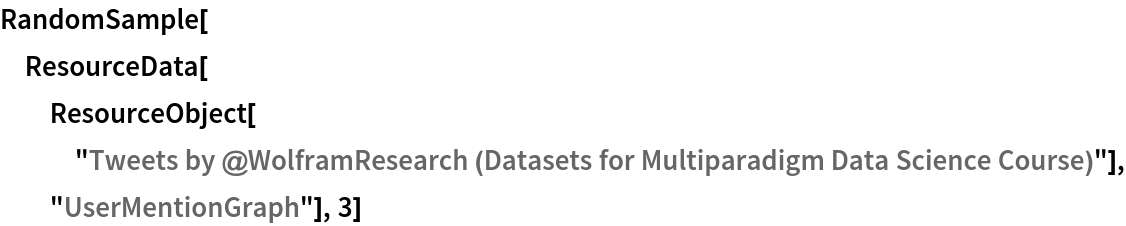Out=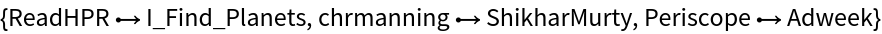### Visualizations

Visualize a DateHistogram of tweets by Wolfram research:

 In:=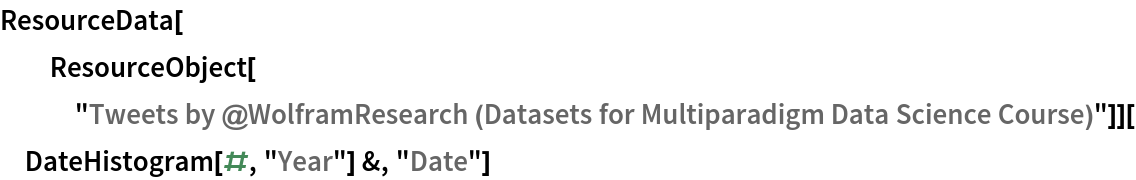Out=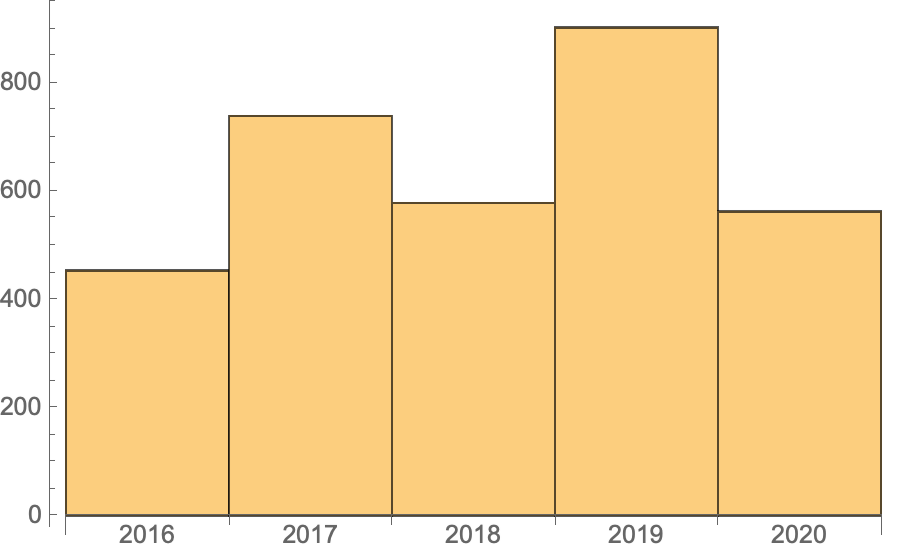Visualize a WordCloud of other users mentioned in the tweets by WolframResearch:

 In:=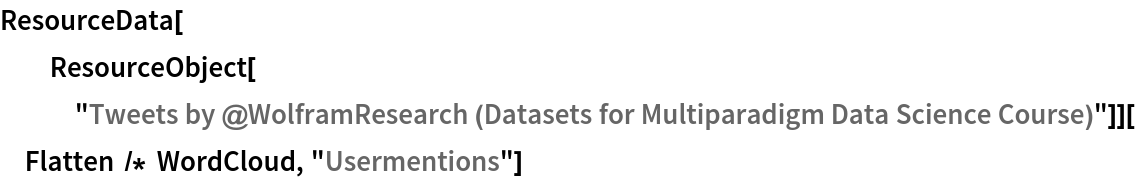Out=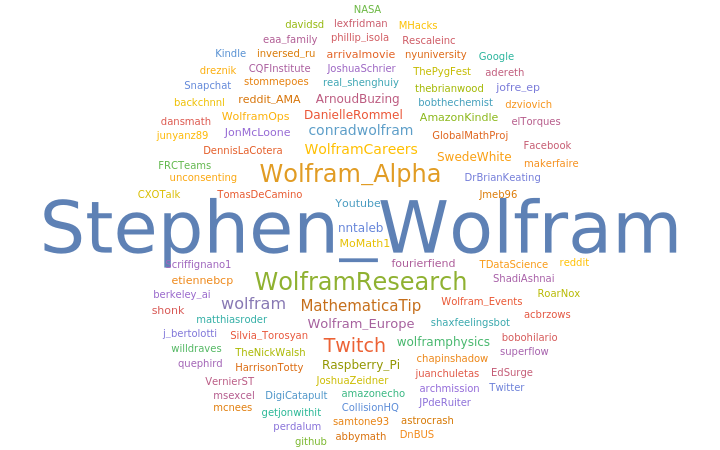Visualize the locations of the users mentioned in the tweets by WolframResearch:

 In:=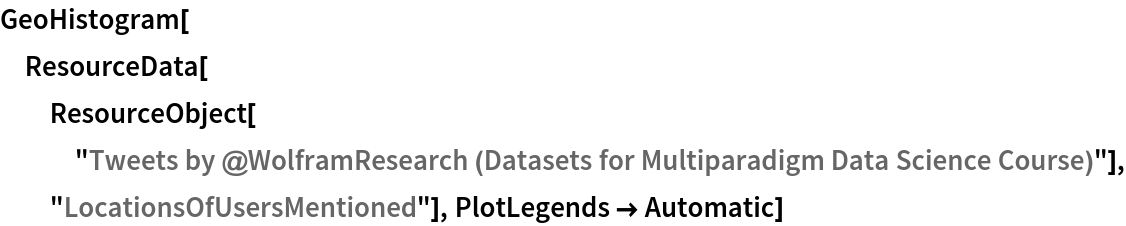Out=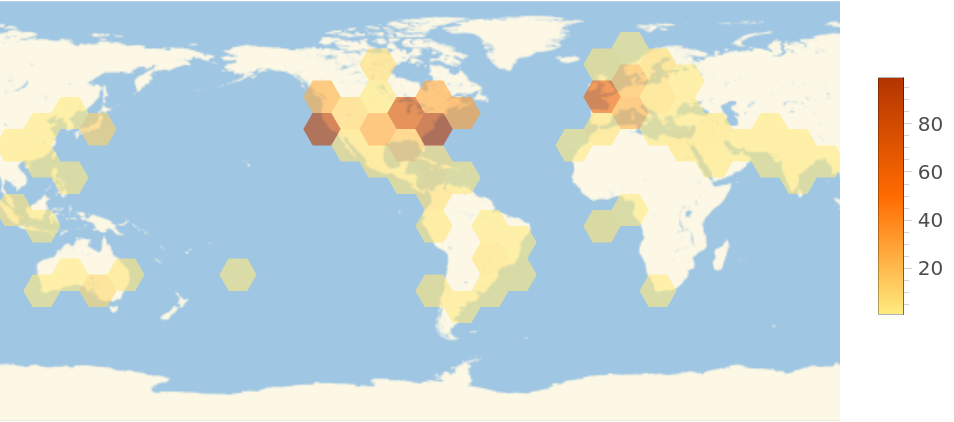Visualize a WordCloud of the hashtags used by these users:

 In:=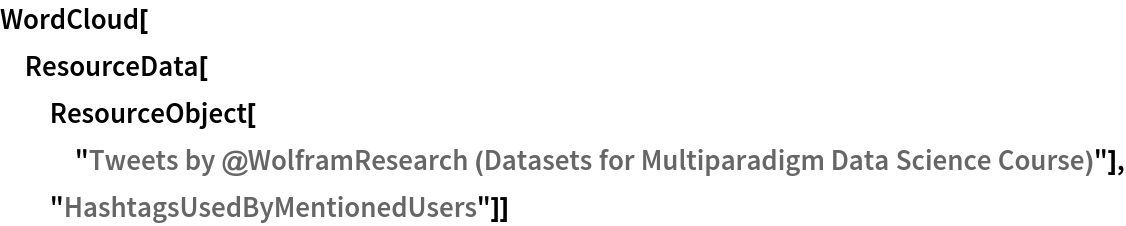Out=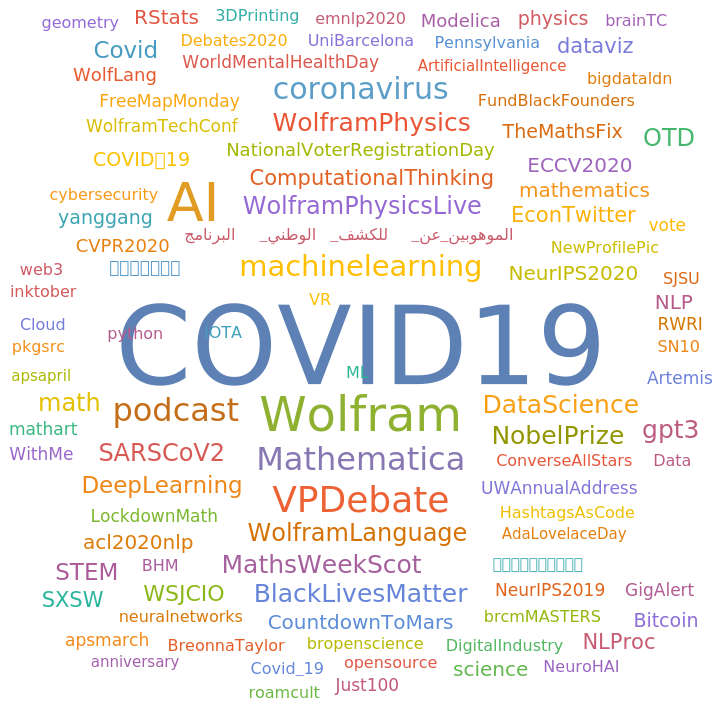### Analysis

Visualize users mentioning each other in their tweets in a graph:

 In:=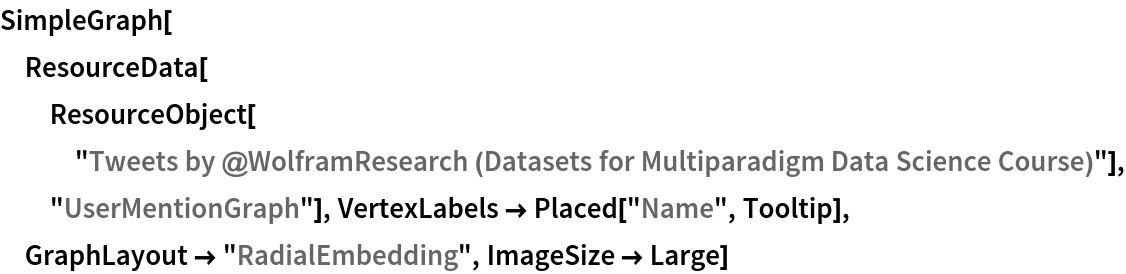Out=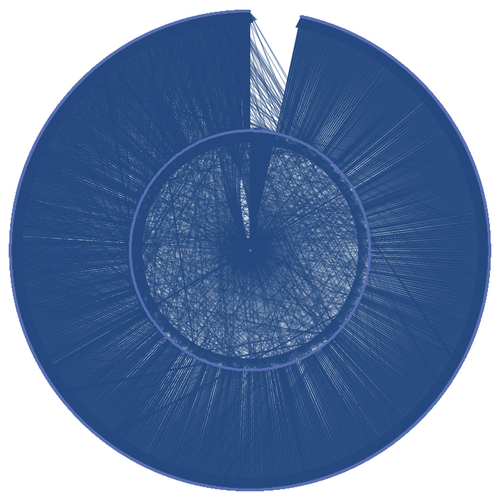Wolfram U, "Tweets by @WolframResearch (Datasets for Multiparadigm Data Science Course)" from the Wolfram Data Repository (2020)